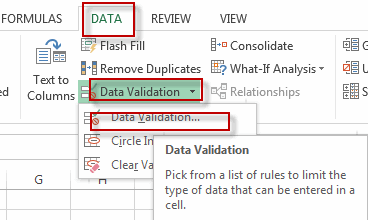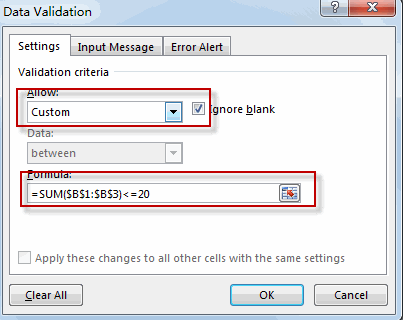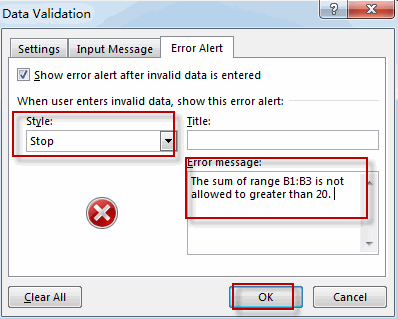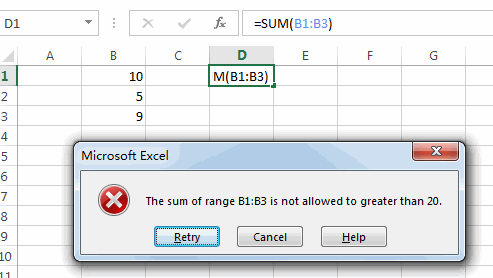# Using Data Validation to Limit Calculated Cell

This post explains that how to limit calculated cell using Data Validation in excel. You can use the Data Validation function to limit what a user can type in a cell. And you can also use the data validation to limit user to whole number, dates, a list of selections or a specific range of values.

For example, there is a cell D1 that has the formula =SUM(B1:B3), if the sum of B1:B3 is greater than 20, then you want excel to pop up a warning message dialog and stop entering value in the range B1:B3. How to do this? You can use the Data Validation to set the criteria to limit this calculated cell using the formula =SUM(\$B\$1:\$B\$3)<=20 in the Data Validation dialog box.

## Using Data Validation to Limit Calculated Cell

To Use Data Validation to limit Data Entry in calculated cell, you can follow these steps:

#1 select the calculated cells that you want to limit.

#2 go to DATA tab, click Data Validation command under Data Tools group, then select Data Validation… menu from the drop down menu list. The Data Validation dialog will appear.#3 select Custom from the drop-down list box of Allow, then type the formula =SUM(\$B\$1:\$B\$3)<=20 into the Formula text box. Click OK.#4 switch to Error Alert tab in the Data Validation dialog box, select stop style from the drop-down list of style. Then you can enter the error warning message in the text box of Error message. Click OK.#5 if the sum of the range B1:B3 is greater than 20, the warning dialog will pop-up.### Related Functions

• Excel SUM function
The Excel SUM function will adds all numbers in a range of cells and returns the sum of these values. You can add individual values, cell references or ranges in excel.The syntax of the SUM function is as below:= SUM(number1,[number2],…)…